## Wednesday, 22 May 2013

### More Conformal Maps With OpenCV

So I finally got the conformal maps code to work with some of the  other functions and the results are quite interesting and strange. Not really what I expected to get. The transformed images vaugely resemble the ones in my math textbook but don't match them exactly.

So again, here's the original image for reference:

I then applied the transformation $w = z^3$ to the image.

The results are quite satisfactory. No surprises there.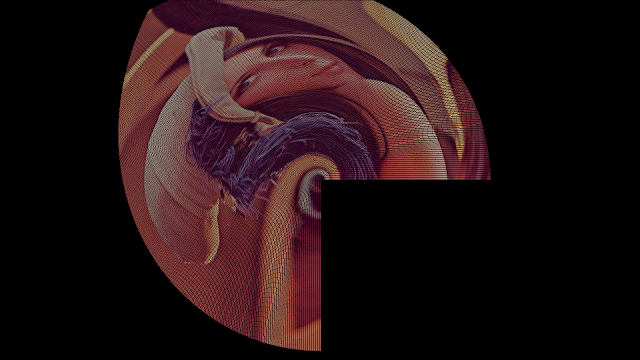$w = z^3$ transformation
Next I tried $w = z + \frac{1}{z}$. I didn't really get what I expected ...$w = z + \frac{1}{z}$
$w = log(z)$ looks like someone flattened the thing along the third dimension.$w = log(z)$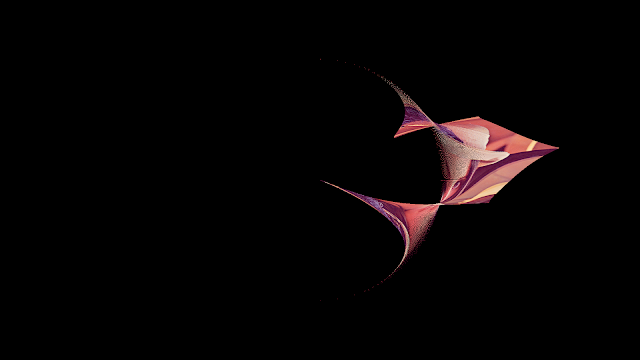Another version of $w = log(z)$ with the image in a different position.
The results for $w = sin(z)$ and $w = e^{z}$ were just plain weird.$w = sin(z)$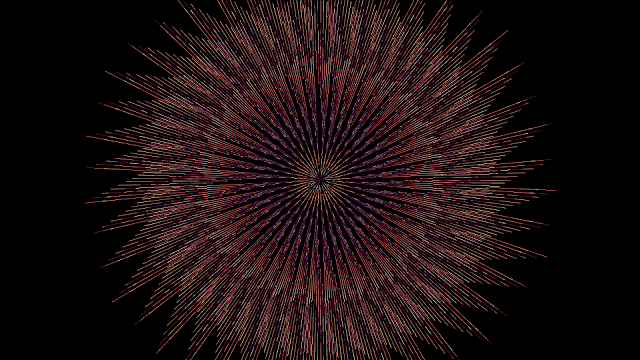$w = e^z$
But my favourite one was the transform $w = tan(z)$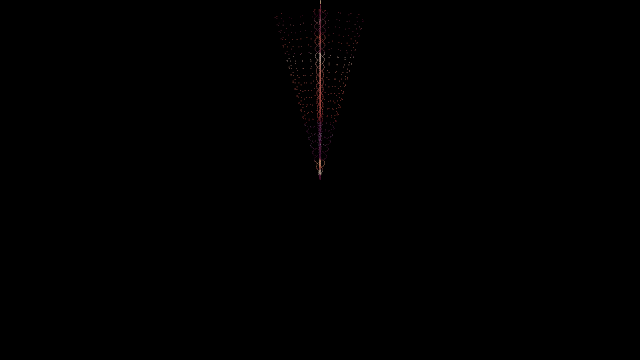$w = tan(z)$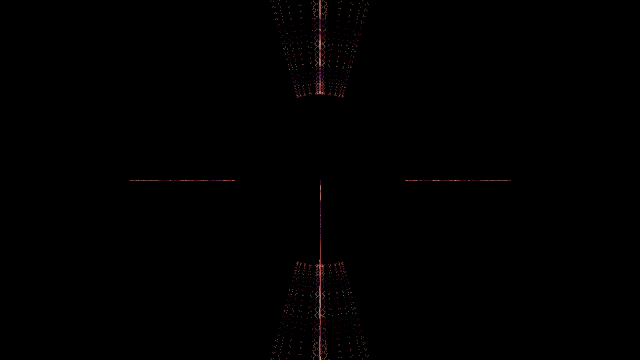$w = tan(z)$ with the image in a different starting position
A perfect example of mathematics creating art. They're not as good as fractals but they're still pretty good.